Tamil Nadu Board of Secondary EducationSSLC (English Medium) Class 6th

# Tamil Nadu Board Samacheer Kalvi solutions for Class 6th Mathematics Term 1 Answers Guide chapter 4 - Geometry [Latest edition]

#### Chapters## Chapter 4: Geometry

Exercise 4.1Exercise 4.2Exercise 4.3Exercise 4.4
Exercise 4.1 [Page 71]

### Tamil Nadu Board Samacheer Kalvi solutions for Class 6th Mathematics Term 1 Answers Guide Chapter 4 GeometryExercise 4.1 [Page 71]

#### Fill in the blanks

Exercise 4.1 | Q 1. (i) | Page 71

A line through two end points ‘A’ and ‘B’ is denoted by ______________

Exercise 4.1 | Q 1. (ii) | Page 71

A line segment from point ‘B’ to point ‘A’ is denoted by _____________

Exercise 4.1 | Q 1. (iii) | Page 71

A ray has ______________ end point(s)

Exercise 4.1 | Q 2 | Page 71

How many line segments are there in the given line? Name them.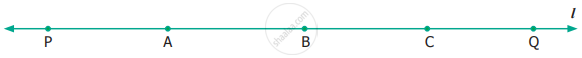Exercise 4.1 | Q 3 | Page 71

Measure the following line segments.Exercise 4.1 | Q 4. (i) | Page 71

Construct a line segment using ruler and compass.

AB = 7.5 cm

Exercise 4.1 | Q 4. (ii) | Page 71

Construct a line segment using ruler and compass.

CD = 3.6 cm

Exercise 4.1 | Q 4. (iii) | Page 71

Construct a line segment using ruler and compass.

QR = 10 cm

Exercise 4.1 | Q 5 | Page 71

From the given figure, name the
(i) parallel lines
(ii) intersecting lines
(iii) points of intersection.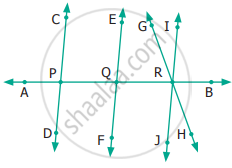Exercise 4.1 | Q 6 | Page 71

From the given figure, name
(i) all pairs of parallel lines.
(ii) all pairs of intersecting lines.
(iii) pair of lines whose point of intersection is ‘V’.
(iv) point of intersection of the lines ‘l2‘ and ‘l3‘.
(v) point of intersection of the lines ‘l1‘, and ‘l5‘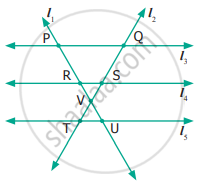#### Objective Type Questions

Exercise 4.1 | Q 7 | Page 71

The number of line segments in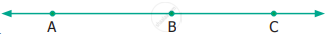is

• 1

• 2

• 3

• 4

Exercise 4.1 | Q 8 | Page 71

A line is denoted as

• AB

• $\overrightarrow{AB}$

• $\overleftrightarrow{AB}$

• bar("AB")

Exercise 4.2 [Pages 79 - 82]

### Tamil Nadu Board Samacheer Kalvi solutions for Class 6th Mathematics Term 1 Answers Guide Chapter 4 GeometryExercise 4.2 [Pages 79 - 82]

Exercise 4.2 | Q 1. (i) | Page 79

Use any number of the given dots to make different angles.

An Acute Angle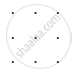Exercise 4.2 | Q 1.(ii) | Page 79

Use any number of the given dots to make different angles.

An Obtuse Angle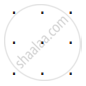Exercise 4.2 | Q 1. (iii) | Page 79

Use any number of the given dots to make different angles.

A Right Angle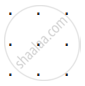Exercise 4.2 | Q 1. (iv) | Page 79

Use any number of the given dots to make different angles.

A Straight Angle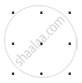Exercise 4.2 | Q 2. (i) | Page 80

Name the vertex and sides from the angle.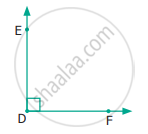Vertex _____________

Sides ______________

Exercise 4.2 | Q 2. (ii) | Page 80

Name the vertex and sides from the angle.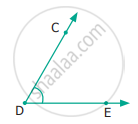Vertex _____________

Sides ______________

Exercise 4.2 | Q 3 | Page 80

Pick out the Right angle from the given figures.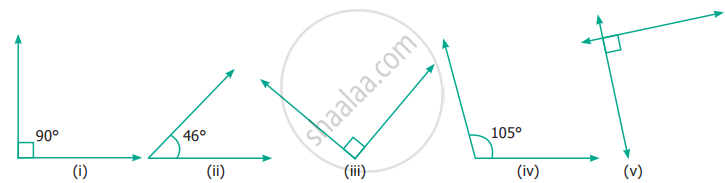Exercise 4.2 | Q 4 | Page 80

Pick out the Acute angle from the given figures.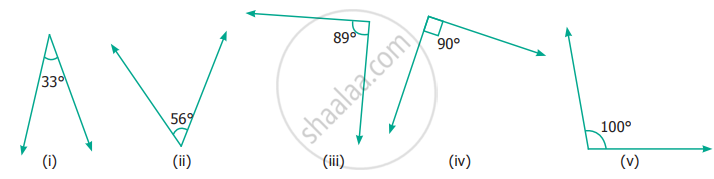Exercise 4.2 | Q 5 | Page 80

Pick out the Obtuse angle from the given figures.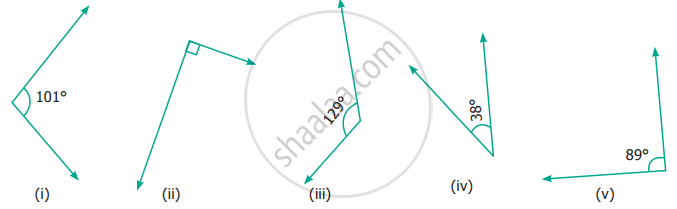Exercise 4.2 | Q 6. (i) | Page 80

Name the angle in the figure given below in all the possible ways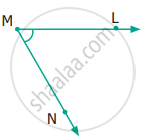Exercise 4.2 | Q 6. (ii) | Page 80

Name the angle in the figure given below in all the possible ways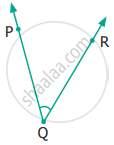Exercise 4.2 | Q 6. (iii) | Page 80

Name the angle in the figure given below in all the possible ways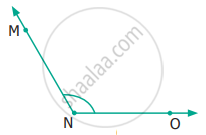Exercise 4.2 | Q 6. (iv) | Page 80

Name the angle in the figure given below in all the possible ways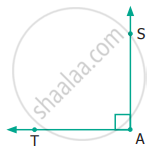#### Say True or False

Exercise 4.2 | Q 7. (i) | Page 81

20° and 70° are complementary

• True

• False

Exercise 4.2 | Q 7. (ii) | Page 81

88° and 12° are complementary

• True

• False

Exercise 4.2 | Q 7. (iii) | Page 81

80° and 180° are supplementary

• True

• False

Exercise 4.2 | Q 7. (iv) | Page 81

0° and 180° are supplementary

• True

• False

Exercise 4.2 | Q 8 (i) | Page 81

Draw and label of the angle.

∠NAS = 90°

Exercise 4.2 | Q 8. (ii) | Page 81

Draw and label of the angle.

∠BIG = 35°

Exercise 4.2 | Q 8. (iii) | Page 81

Draw and label of the angle.

∠SMC = 145°

Exercise 4.2 | Q 8. (iv) | Page 81

Draw and label of the angle

∠ABC = 180°

Exercise 4.2 | Q 9. (i) | Page 81

Identify the type of angle shown by the hands of the given clock.Exercise 4.2 | Q 9. (ii) | Page 81

Identify the type of angle shown by the hands of the given clock.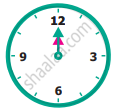Exercise 4.2 | Q 9. (iii) | Page 81

Identify the type of angle shown by the hands of the given clock.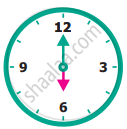Exercise 4.2 | Q 9. (iv) | Page 81

Identify the type of angle shown by the hands of the given clock.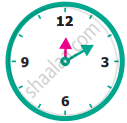Exercise 4.2 | Q 9. (v) | Page 81

Identify the type of angle shown by the hands of the given clock.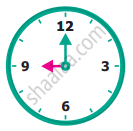Exercise 4.2 | Q 10. (i) | Page 81

Find the complementary angle of 30°

Exercise 4.2 | Q 10. (ii) | Page 81

Find the complementary angle of 26°

Exercise 4.2 | Q 10. (iii) | Page 81

Find the complementary angle of 85°

Exercise 4.2 | Q 10. (iv) | Page 81

Find the complementary angle of 0°

Exercise 4.2 | Q 10. (v) | Page 81

Find the complementary angle of 90°

Exercise 4.2 | Q 11. (i) | Page 81

Find the supplementary angle of 70°

Exercise 4.2 | Q 11. (ii) | Page 81

Find the supplementary angle of 35°

Exercise 4.2 | Q 11. (iii) | Page 81

Find the supplementary angle of 165°

Exercise 4.2 | Q 11. (iv) | Page 81

Find the supplementary angle of 90°

Exercise 4.2 | Q 11. (v) | Page 81

Find the supplementary angle of 0°

Exercise 4.2 | Q 12. (i) | Page 81

Find the supplementary/complementary angle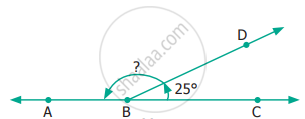Exercise 4.2 | Q 12. (ii) | Page 81

Find the supplementary/complementary angle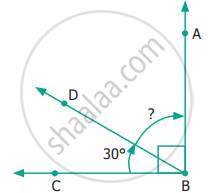Exercise 4.2 | Q 12. (iii) | Page 81

Find the supplementary/complementary angle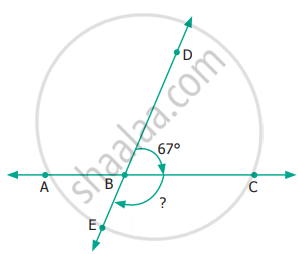Exercise 4.2 | Q 12. (iv) | Page 81

Find the supplementary/complementary angle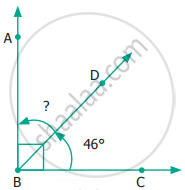#### Objective Type Questions

Exercise 4.2 | Q 13 | Page 82

In this Figure, which is not the correct way of naming an angle?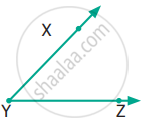• ∠Y

• ∠ZXY

• ∠ZYX

• ∠XYZ

Exercise 4.2 | Q 14 | Page 82

In this Figure, ∠AYZ = 45°. If point ‘A’ is shifted to point ‘B’ along the ray, then the measure of ∠BYZ is ____________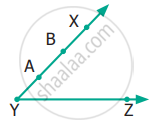• more than 45°

• 45°

• less than 45°

• 90°

Exercise 4.3 [Pages 83 - 84]

### Tamil Nadu Board Samacheer Kalvi solutions for Class 6th Mathematics Term 1 Answers Guide Chapter 4 GeometryExercise 4.3 [Pages 83 - 84]

Exercise 4.3 | Q 1. (i) | Page 83

Observe the diagram and fill in the blank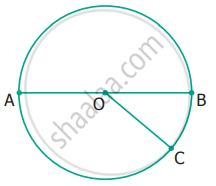‘A’, ‘O’ and ‘B’ are _________ points.

Exercise 4.3 | Q 1. (ii) | Page 83

Observe the diagram and fill in the blank‘A’, ‘O’ and ‘C’ are _________ points

Exercise 4.3 | Q 1. (iii) | Page 83

Observe the diagram and fill in the blank‘A’, ‘B’ and ‘C’ are _________ points

Exercise 4.3 | Q 1. (iv) | Page 83

Observe the diagram and fill in the blank_________ is the point of concurrency

Exercise 4.3 | Q 2 | Page 83

Draw any line and mark any 3 points that are collinear

Exercise 4.3 | Q 3 | Page 83

Draw any line and mark any 4 points that are not collinear

Exercise 4.3 | Q 4 | Page 83

Draw any 3 lines to have a point of concurrency

Exercise 4.3 | Q 5 | Page 83

Draw any 3 lines that are not concurrent. Find the number of points of intersection

#### Objective Type Questions

Exercise 4.3 | Q 6 | Page 84

A set of collinear points in the figure are ______________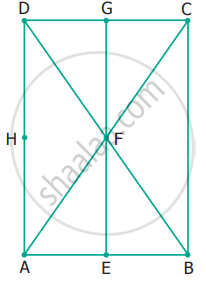• A, B, C

• A, F, C

• B, C, D

• A, C, D

Exercise 4.3 | Q 7 | Page 84

A set of non-collinear points in the figure are ___________• A, F, C

• B, F, D

• E, F, G

• A, D, C

Exercise 4.3 | Q 8 | Page 84

A point of concurrency in the figure is ____________• E

• F

• G

• H

Exercise 4.4 [Pages 84 - 86]

### Tamil Nadu Board Samacheer Kalvi solutions for Class 6th Mathematics Term 1 Answers Guide Chapter 4 GeometryExercise 4.4 [Pages 84 - 86]

#### Miscellaneous Practice Problems

Exercise 4.4 | Q 1. (i) | Page 84

Find the type of lines marked in thick lines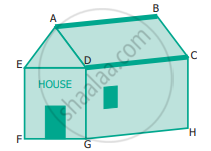• Parallel

• intersecting

• perpendicular

Exercise 4.4 | Q 1. (ii) | Page 84

Find the type of lines marked in thick lines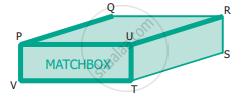• Parallel

• intersecting

• perpendicular

Exercise 4.4 | Q 1. (iii) | Page 84

Find the type of lines marked in thick lines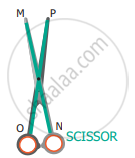• Parallel

• intersecting

• perpendicular

Exercise 4.4 | Q 2 | Page 84

Find the parallel and intersecting line segments in the picture given below.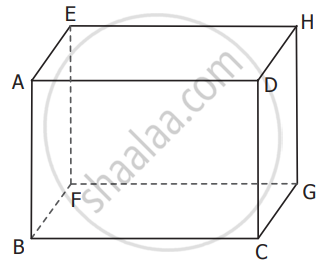Exercise 4.4 | Q 3 | Page 84

Name the following angle as shown in the figure.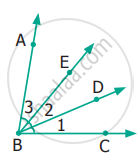(i) ∠1 =
(ii) ∠2 =
(iii) ∠3 =
(iv) ∠1 + ∠2 =
(v) ∠2 + ∠3 =
(vi) ∠1 + ∠2 + ∠3 =

Exercise 4.4 | Q 4. (i) | Page 85

Measure the angle of the given figure using protractor and identify the type of angle as acute, obtuse, right or straight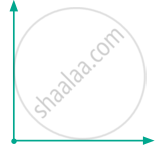Exercise 4.4 | Q 4. (ii) | Page 85

Measure the angle of the given figure using the protractor and identify the type of angle as acute, obtuse, right or straight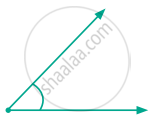Exercise 4.4 | Q 4. (iii) | Page 85

Measure the angle of the given figure using the protractor and identify the type of angle as acute, obtuse, right or straight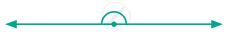Exercise 4.4 | Q 4. (iv) | Page 85

Measure the angle of the given figure using the protractor and identify the type of angle as acute, obtuse, right or straight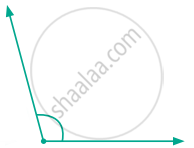Exercise 4.4 | Q 5. (i) | Page 85

From the figure given below, classify the following pair of angles into complementary and non complementary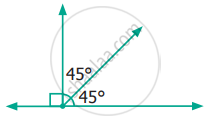Exercise 4.4 | Q 5. (ii) | Page 85

From the figure given below, classify the following pair of angles into complementary and non complementary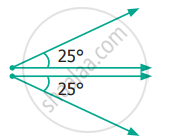Exercise 4.4 | Q 5. (iii) | Page 85

From the figure given below, classify the following pair of angles into complementary and non complementary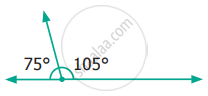Exercise 4.4 | Q 5. (iv) | Page 85

From the figure given below, classify the following pair of angles into complementary and non complementary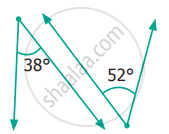Exercise 4.4 | Q 6. (i) | Page 85

From the figure given below, classify the following pair of angles into supplementary and non supplementary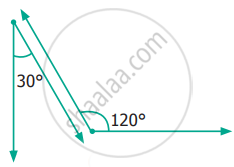Exercise 4.4 | Q 6. (ii) | Page 85

From the figure given below, classify the following pair of angles into supplementary and non supplementary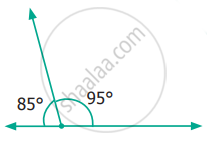Exercise 4.4 | Q 6. (iii) | Page 85

From the figure given below, classify the following pair of angles into supplementary and non supplementary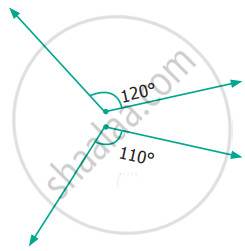Exercise 4.4 | Q 6. (iv) | Page 85

From the figure given below, classify the following pair of angles into supplementary and non supplementary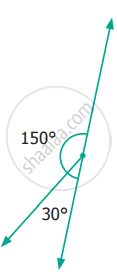Exercise 4.4 | Q 7. (i) | Page 85

From the figure name a pair of complementary angles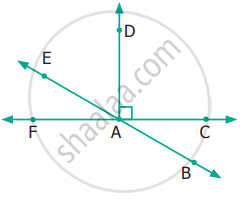Exercise 4.4 | Q 7. (ii) | Page 85

From the figure name a pair of supplementary angles#### Challenging Problems

Exercise 4.4 | Q 8 | Page 86

Think and write an object having

• Parallel lines (1) __________ (2) __________ (3) __________
• Perpendicular lines (1) __________ (2) __________ (3) __________
• Intersecting lines (1) __________ (2) __________ (3) __________
Exercise 4.4 | Q 9 | Page 86

Which angle is equal to twice its complement?

Exercise 4.4 | Q 10 | Page 86

Which angle is equal to two-third of its supplement?

Exercise 4.4 | Q 11 | Page 86

Given two angles are supplementary and one angle is 20° more than other. Find the two angles

Exercise 4.4 | Q 12 | Page 86

Two complementary angles are in ratio 7 : 2. Find the angles

Exercise 4.4 | Q 13 | Page 86

Two supplementary angles are in ratio 5 : 4. Find the angles

## Chapter 4: Geometry

Exercise 4.1Exercise 4.2Exercise 4.3Exercise 4.4## Tamil Nadu Board Samacheer Kalvi solutions for Class 6th Mathematics Term 1 Answers Guide chapter 4 - Geometry

Tamil Nadu Board Samacheer Kalvi solutions for Class 6th Mathematics Term 1 Answers Guide chapter 4 (Geometry) include all questions with solution and detail explanation. This will clear students doubts about any question and improve application skills while preparing for board exams. The detailed, step-by-step solutions will help you understand the concepts better and clear your confusions, if any. Shaalaa.com has the Tamil Nadu Board of Secondary Education Class 6th Mathematics Term 1 Answers Guide solutions in a manner that help students grasp basic concepts better and faster.

Further, we at Shaalaa.com provide such solutions so that students can prepare for written exams. Tamil Nadu Board Samacheer Kalvi textbook solutions can be a core help for self-study and acts as a perfect self-help guidance for students.

Concepts covered in Class 6th Mathematics Term 1 Answers Guide chapter 4 Geometry are Introduction of Geometry, Concept of Line, Concept of Line Segment, Measuring Line Segments, Concept of Ray, Concept of Angle - Arms, Vertex, Interior and Exterior Region, Measuring Angles, Angles of Special Measures - 30°, 45°, 60°, 90°, and 120°, Complementary Angles, Supplementary Angles, Types of Angles- Acute, Obtuse, Right, Straight, Reflex, Complete and Zero Angle., Points and Lines.

Using Tamil Nadu Board Samacheer Kalvi Class 6th solutions Geometry exercise by students are an easy way to prepare for the exams, as they involve solutions arranged chapter-wise also page wise. The questions involved in Tamil Nadu Board Samacheer Kalvi Solutions are important questions that can be asked in the final exam. Maximum students of Tamil Nadu Board of Secondary Education Class 6th prefer Tamil Nadu Board Samacheer Kalvi Textbook Solutions to score more in exam.

Get the free view of chapter 4 Geometry Class 6th extra questions for Class 6th Mathematics Term 1 Answers Guide and can use Shaalaa.com to keep it handy for your exam preparation